Share
Any question? Call us at : +33 970 405 020
THE ART OF AFRICAN CRAFTS QUALITY
0
item
0.00 € VAT Incl.
Your shopping cart
Products search Advanced search

To know the ring size ?

We have our own international classification system size: the 'size Nanadiany' '(N). This system covers all sizes of rings around the world. Refer to the system in your country, a little lower, and take note of the equivalent of the size of your home country Nanadiany (N) to place your order
French and European size
By means of this board chose the equivalent of your French or European Size at Nanadiany.

Nanadiany size = French or European Size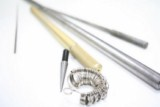USA and Canada size
By means of this board chose the equivalent of your USA and Canada Size at Nanadiany.

Nanadiany size = USA and Canada Size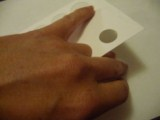English ( UK) size
By means of this board chose the equivalent of your English (UK) Size at Nanadiany.

Nanadiany size = English (UK) Size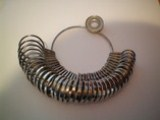German size
By means of this board chose the equivalent of your German Size at Nanadiany.

Nanadiany size = German Size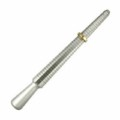Nanadiany size with the internal ring diameter in mm
o check the size: if you already have a ring that fits you perfectly, measure in the internal diameter and consult the table. You will have the number that corresponds to the size of your ring. The number you have chosen is one that must be given by a command.
Note: if indeed received the ring is too large, try to put one or more layers of nail polish (colorless or otherwise) within the ring to thicken a little. Or switch finger ... ;-)

Size Nanadiany = internal diameter of the ring in mm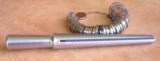N1 = 11.0 N2 = 12.1 N3 = 12.3 N4 = 12.6 N5 = 12.7 N6 = 13.0 N7 = 13.2 N8 = 13.4 N9 = 13.6 N10 = 13.8 N11 = 14.0 N12 = 14.1 N13 = 14.3 N15 = 14.5 N16 = 14.6 N17 = 14.8 N18 = 15.0 N19 = 15.2 N20 = 15.4 N49A = 15.7 N50= 15.8 N50A = 16.0 N50B = 16.1 N51 = 16.4 N51A = 16.5 N52 = 16.6 N52B = 16.7 N53 = 16.8 N53A = 17.0 N53B = 17.1 N54 = 17.2 N54A = 17.3 N55 = 17.4 N55A = 17.6 N56 = 17.7 N56A = 18.0 N57 = 18.1 N57A = 18.3 N58 = 18.5 N58A = 18.6 N59 = 18.8 N59A = 19.0 N60 = 19.1 N60A = 19.3 N61 = 19.5 N61A = 19.6 N62 = 19.8 N62A = 20.0 N63 = 20.0 N63A = 20.2 N64 = 20.3 N64A= 20.7 N65 = 20.8 N65A = 20.9 N65B = 21.1 N66 = 21.2  N67 =21.3 N68 = 21.6 N69 = 22 N70 = 22.3